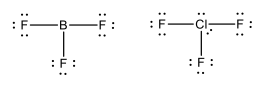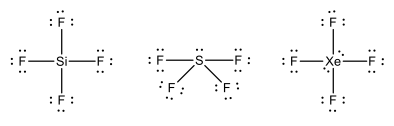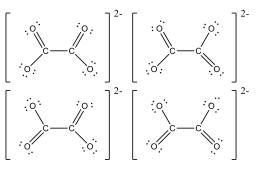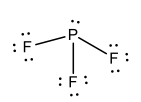Study Guide

# Types of Bonds and Orbitals Questions

## Types of Bonds and Orbitals Questions

1. Using Lewis dot structures, explain why the ClF3 molecule is polar while the BF3 molecule is non-polar.

2. Which of the following tetrafluorides is non-polar? Use Lewis dot structures to explain your findings.

SiF4, SF4, XeF4

3. Use Lewis dot structure to show why the carbon-oxygen bonds in the oxalate ion (C2O42-) are all equal.

4. Draw the Lewis dot structure for PF3 and predict the molecular geometry.

5. Is the PF3 molecule from the previous question polar or non-polar? Explain.

6. Explain how to draw the Lewis dot structure of an ion.

7. What are the electron configurations for Cl and Cl-?
8. Consider an arsenic atom. What are the four quantum numbers for each of the two electrons in the 4s orbital?

9. How many unpaired electrons are in a Co3+ ion and explain your reasoning.

10. Explain the difference between ionic compounds and covalently bonded compounds and give an example of each.

1. Using Lewis dot structures, explain why the ClF3 molecule is polar while the BF3 molecule is non-polar.The BF3 has three bonding pairs and zero non-bonding pairs of electrons on the central atom. This makes the molecule non-polar. The ClF3 has five pairs of electrons around the central atom, two of which are lone pairs. This makes the molecule polar.

2. Which of the following tetrafluorides is non-polar? Use Lewis dot structures to explain your findings.
SiF4, SF4, XeF4Silicon tetrafluoride is the only one of the compounds that is non-polar because there are no lone pairs on the central atom.

3. Use Lewis dot structure to show why the carbon-oxygen bonds in the oxalate ion (C2O42-) are all equal.The above resonance structures may be drawn for the oxalate ion. The presence of resonance structures equalizes the bonds and makes them all the same length.

4. Draw the Lewis dot structure for PF3 and predict the molecular geometry.Trigonal pyramidal. Notice the pair of electrons.

5. Is the PF3 molecule from the previous question polar or non-polar? Explain.

The PF3 molecule is polar. The lone pair of electrons on the P atom leads to an unsymmetrical distribution of charge.

6. Explain how to draw the Lewis dot structure of an ion.

If it's a negative ion, add the negative charge to the valence electron count. If it's a positive ion, subtract the positive charge from the valance electron count. In either case put brackets [ ] around the entire structure, and write the charge outside of the brackets.

7. What are the electron configurations for Cl and Cl-?

Cl: 1s22s22p63s23p5Cl-: 1s22s22p63s23p6

8. Consider an arsenic atom. What are the four quantum numbers for each of the two electrons in the 4s orbital?

4, 0, 0, +1/2
4, 0, 0, -1/2

9. How many unpaired electrons are in a Co+3 ion and explain your reasoning.

There are 3 unpaired electrons because

4s(//) 3d (//) (//) (/ ) (/) (/ )

10. Explain the difference between ionic compounds and covalently bonded compounds and give an example of each.

Ionic Compounds are bonds between a metal and a non-metal. Electrons are transferred from metal to non-metal in ionic bonds. An example would be NaCl. Covalent bonds are bonds between two non-metals. Electrons are shared in covalent bonds. An example would be CH4.